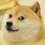# Linear Mean Function

I've been looking at functions $$f:\mathbb{R}^n \to \mathbb{R}$$ which necessarily satisfy the following 3 properties. Given $$a_1, a_2, \dots a_n \in \mathbb{R}^+$$

$\begin{array} { l l } 1. & f(x_1 + c, x_2 + c, \dots , x_n + c) = f(x_1, x_2, \dots , x_n) + c \\ 2. & f(cx_1, cx_2, \dots , cx_n) = cf(x_1, x_2, \dots, x_n) \\ 3. & \sum_{i=1}^n a_i x_i = 0 \Leftrightarrow f(x_1, x_2, \dots, x_3) = 0 \end{array}$

I believe that the the only function that satisfies this is $f(x_1,x_2, \dots, x_n) = \frac{\sum_{i=1}^n a_i x_i}{\sum_{i=1}^n a_i}$

I have the proof too while I'll write up later. I'm interested in all of your interpretations of this.

What happens if we only have 2 out of these 3 conditions?Note by Josh Banister
4 years, 6 months ago

This discussion board is a place to discuss our Daily Challenges and the math and science related to those challenges. Explanations are more than just a solution — they should explain the steps and thinking strategies that you used to obtain the solution. Comments should further the discussion of math and science.

When posting on Brilliant:

• Use the emojis to react to an explanation, whether you're congratulating a job well done , or just really confused .
• Ask specific questions about the challenge or the steps in somebody's explanation. Well-posed questions can add a lot to the discussion, but posting "I don't understand!" doesn't help anyone.
• Try to contribute something new to the discussion, whether it is an extension, generalization or other idea related to the challenge.
• Stay on topic — we're all here to learn more about math and science, not to hear about your favorite get-rich-quick scheme or current world events.

MarkdownAppears as
*italics* or _italics_ italics
**bold** or __bold__ bold
- bulleted- list
• bulleted
• list
1. numbered2. list
1. numbered
2. list
Note: you must add a full line of space before and after lists for them to show up correctly
paragraph 1paragraph 2

paragraph 1

paragraph 2

[example link](https://brilliant.org)example link
> This is a quote
This is a quote
    # I indented these lines
# 4 spaces, and now they show
# up as a code block.

print "hello world"
# I indented these lines
# 4 spaces, and now they show
# up as a code block.

print "hello world"
MathAppears as
Remember to wrap math in $$ ... $$ or $ ... $ to ensure proper formatting.
2 \times 3 $2 \times 3$
2^{34} $2^{34}$
a_{i-1} $a_{i-1}$
\frac{2}{3} $\frac{2}{3}$
\sqrt{2} $\sqrt{2}$
\sum_{i=1}^3 $\sum_{i=1}^3$
\sin \theta $\sin \theta$
\boxed{123} $\boxed{123}$

## Comments

Sort by:

Top Newest

Let $x_1$, $x_2$, $\dots$, $x_n$ be given real numbers. We seek a value of $c$ so that $a_1 (x_1 + c) + a_2 (x_2 + c) + \dots + a_n (x_n + c) = 0.$ Solving for $c$, we get $c = -\frac{a_1 x_1 + a_2 x_2 + \dots + x_n x_n}{a_1 + a_2 + \dots + a_n}.$

From the third property, $f(x_1 + c, x_2 + c, \dots, x_n + c) = 0$, so by the first property, $f(x_1, x_2, \dots, x_n) = -c = \frac{a_1 x_1 + a_2 x_2 + \dots + x_n x_n}{a_1 + a_2 + \dots + a_n}.$ Note that the second property is never used.

- 4 years, 6 months ago

Log in to reply

The idea behind the second property is what happens if the third one was never there? (What happens now if we only assume the first 2 ideas. Hence the linear part of the title)

- 4 years, 6 months ago

Log in to reply

That's very interesting! I believe there is a geometric interpretation of the result, as the (normalized) distance of the vector x projected onto a.

Staff - 4 years, 6 months ago

Log in to reply

×

Problem Loading...

Note Loading...

Set Loading...